#### Computing the boundary of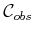So far, the method quickly identifies each edge that contributes to. It can also construct a solid representation ofin terms of half-planes. This requires defining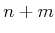linear equations (assuming there are no collinear edges).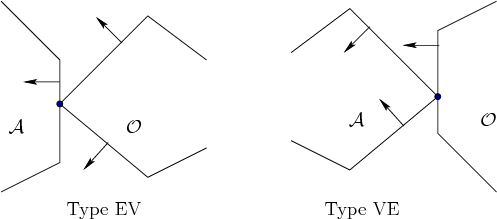There are two different ways in which an edge ofis generated, as shown in Figure 4.16 [282,657]. Type EV contact refers to the case in which an edge of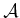is in contact with a vertex of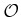. Type EV contacts contribute toedges of, once for each edge of. Type VE contact refers to the case in which a vertex ofis in contact with an edge of. This contributes to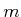edges of. The relationships between the edge normals are also shown in Figure 4.16. For Type EV, the inward edge normal points between the outward edge normals of the obstacle edges that share the contact vertex. Likewise for Type VE, the outward edge normal ofpoints between the inward edge normals of.

Using the ordering shown in Figure 4.15b, Type EV contacts occur precisely when an edge normal ofis encountered, and Type VE contacts occur when an edge normal ofis encountered. The task is to determine the line equation for each occurrence. Consider the case of a Type EV contact; the Type VE contact can be handled in a similar manner. In addition to the constraint on the directions of the edge normals, the contact vertex ofmust lie on the contact edge of. Recall that convex obstacles were constructed by the intersection of half-planes. Each edge ofcan be defined in terms of a supporting half-plane; hence, it is only necessary to determine whether the vertex oflies on the line through the contact edge of. This condition occurs precisely asand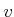are perpendicular, as shown in Figure 4.17, and yields the constraint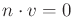.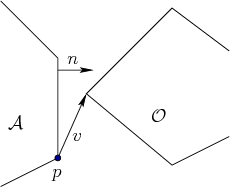Note that the normal vectordoes not depend on the configuration ofbecause the robot cannot rotate. The vector, however, depends on the translation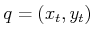of the point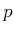. Therefore, it is more appropriate to write the condition as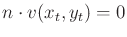. The transformation equations are linear for translation; hence,is the equation of a line in. For example, if the coordinates ofare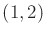for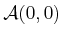, then the expression forat configuration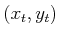is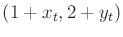. Let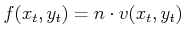. Let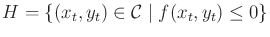. Observe that any configurations not in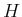must lie in. The half-planeis used to define one edge of. The obstacle regioncan be completely characterized by intersecting the resulting half-planes for each of the Type EV and Type VE contacts. This yields a convex polygon inthat hassides, as expected.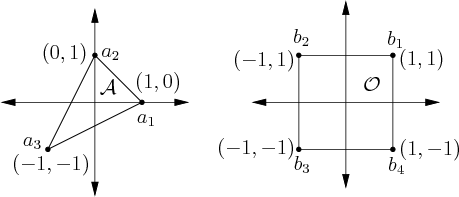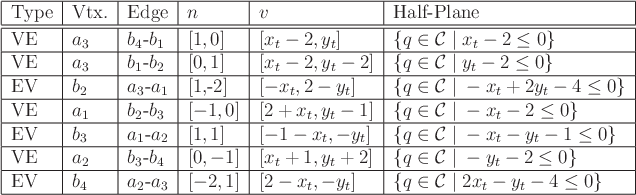Example 4..15 (The Boundary of)   Consider building a geometric model offor the robot and obstacle shown in Figure 4.18. Suppose that the orientation ofis fixed as shown, and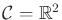. In this case,will be a convex polygon with seven sides. The contact conditions that occur are shown in Figure 4.19. The ordering as the normals appear around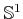(using inward edge normals forand outward edge normals for). Theedges and their corresponding contact types are shown in Figure 4.19.Steven M LaValle 2020-08-14